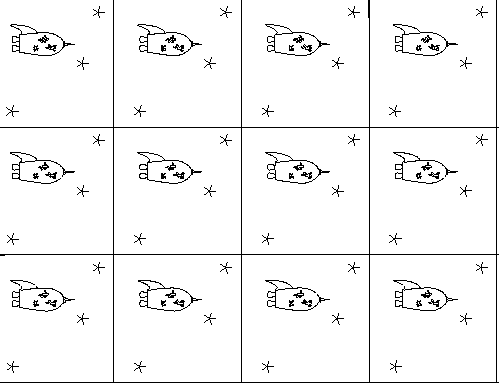### Problem Set 5: Flat Donuts and Round Donuts

You may work in a group of 3 people maximum, or work alone. Each group must write up their work in their own words. The purpose of homework is to learn and practice computational strategies, concepts, and develop critical thinking and problem-solving skills, so you should try problems on your own. Feel free to talk to me or each other if you are stuck on this assignment, but be sure to acknowledge any sources including each other, like "The insight for this solution came from a conversation with Joel." If you know how to do a problem and are asked for help, try to give hints rather than the solution.

Be sure to define all terms you use, draw your own pictures, explain in your own words, give proper reference.

1. ### A Flat Donut

Part A: A flat torus can be theoretically obtained by taking a square and identifying the edges straight across (top to bottom and separately left to right) without distorting the geometry of the interior. So a covering space would be infinitely squares next to each other which are exact copies of each other:How many geodesics join two points in a flat torus? Explain in your own words and through pictures you draw yourself in the covering space.

Part B: First, can a geodesic on a flat torus ever intersect itself by closing back up at a 0 or 180 degree angle? If so give an example and if no, explain. Next, can a geodesic on a flat torus ever intersect itself in angles that differ from integer multiples of pi? If so give an example and if no, explain. Explain in your own words and through pictures you draw yourself in the covering space.

Part C A flat torus cannot be embedded in R3 without distorting the curvature and geodesics in some way (see problem 2). Read Example 5.4.9 beginning on p. 229, which discusses a parametrization of a flat torus in R4. In this parametrization, calculate Xu, Xv, E=Xu*Xu, F=Xu*Xv and G=Xv*Xv [the dot products] by-hand and show work.

Part D: Next write down the metric form (ds/dt)2 and compare it with the Pythagorean theorem to explain why this is called "flat".
2. ### A Round Donut

First read p. 73 Exercise 2.1.13, which gives the parametrization of a round torus Part A: Open up the Maple file on geodesic and normal curvatures that we have been using in class and input a parametrization for a round donut, choosing specific (nonzero) values for r and R. Next find a curve that is NOT a geodesic. Provide your new values for each of the following (these are the commands I used for the sphere) for a curve that is not a geodesic on the round torus:
g := (x,y) -> [cos(x)*sin(y), sin(x)*sin(y), cos(y)]:
a1:=0: a2:=Pi: b1:=0: b2:=Pi:
c1 := 1: c2 := 3:
Point := 2:
f1:= (t) -> t:
f2:= (t) -> 1:

Part B: Sketch by-hand or print out a picture of the curve that is NOT a geodesic, the curvature vectors (label them to clarify which is which) and the torus.

Part C: Now repeat the same process, but find a curve that is a geodesic. Provide your new values for each of the following (these are the commands I used for the sphere) for a curve on a round donut that is a geodesic:
g := (x,y) -> [cos(x)*sin(y), sin(x)*sin(y), cos(y)]:
a1:=0: a2:=Pi: b1:=0: b2:=Pi:
c1 := 1: c2 := 3:
Point := 2:
f1:= (t) -> t:
f2:= (t) -> 1:

Part D: Sketch by-hand or print out a picture of the geodesic, the curvature vectors and the torus.

Part E: Discuss your curves from an intrinsic point of view - ie why is the geodesic a geodesic, and why is the other curve not "straight." You should refer to an intrinsic argument - ie symmetries of your feet, a car that does or does not need to turn the steering wheel, and/or a masking tape or ribbon test.

Part F: Examine the the roles of u and v in the book's parametrization of the donut: hold one constant and explain what kind of curve the other gives and then the reverse.

Part G Use this Maple document on the first fundamental form to input the general round torus (leave r and R as variables), and then to calculate Xu, Xv and Xu x Xv. Write down the results from Maple.

Part H Using Part G, first explain why Xu x Xv is never the 0 vector. Next, what does this tell you about the existence of that tangent plane (does it always, never or only sometimes exist for this parametrization--why?)

Part I: First use Xu and Xv from your Maple work in Part G, to compute F=Xu · Xv by-hand and show work on your dot product computation. Then interpret your result - ie what does it tell you about the relationship between Xu and Xv.

Part J: Use the same Maple document on the first fundamental form to calculate EFG and the determinant and write down what Maple provides.

Part K: Next write down the metric form (ds/dt)2 and compare it with the Pythagorean theorem to show how it differs from a "flat" metric.

Grad Problem: Set up a double integral of the the square root of the determinant of the first fundamental form (see 2.29 on First Fundamental Form) to find the surface area of the torus. Include the limits of integration and then integrate to solve.